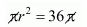# A solid metal sphere of 6 cm diameterQuestion:

A solid metal sphere of 6 cm diameter is melted and a circular sheet of thickness 1 cm is prepared. Determine the diameter of the sheet.

Solution:

Diameter of sphere = 6 cm

Therefore,

Therefore,

Surface area of sphere

$=4 \pi r^{2}$

$=4 \times \pi \times(3)^{2}$

$=36 \pi \mathrm{cm}^{2}$

Area of the circular sheet $=\pi r^{2}$

Therefore,

Surface area of sphere = area of the circular sheet$r=6 \mathrm{~cm}$

Therefore,

Diameter of the sheet = 2 × 6 = 12 cm